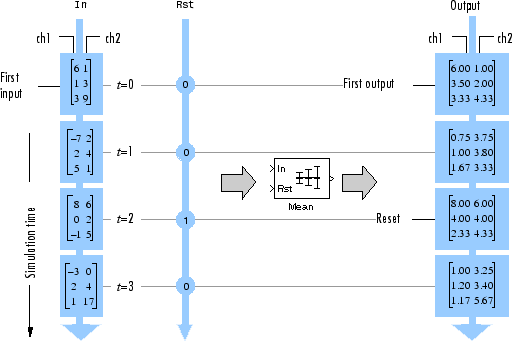# Compute the Running Mean

Compute the running mean of a 3-by-2 matrix input, `dsp_examples_u`, using the Mean block.

Open the model.

```model = 'ex_runningmean_ref'; open_system(model) ```The Input processing parameter is set to `Columns as channels (frame based)`. The block processes the input as a two-channel signal with a frame size of three. The running mean is reset at t = 2 by an impulse to the block's Rst port.

Run the model.

```sim(model) ```In the `Running` mode, the block outputs the mean value over each channel since the last reset. At t = 2, the reset event occurs. The window of data in the second column now contains only 6.

Close the model.

```close_system(model) ```